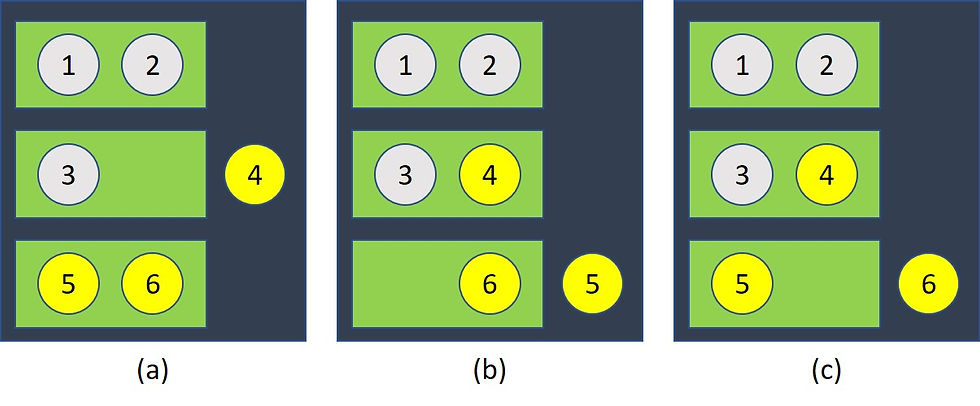top of page
Search

Probability is an integral part of maths and statistics in particular. From calculating the probability of winning a lottery ticket to to predicting the likeliness of a weather event occurring, there are different mathematical ways of calculating the likelihood of something happing. Below are a few examples of some probability paradoxes that aim to explain probabilities in different circumstances.

There are three boxes:

• The first contains two gold coins

• The second contains two silver coins

• The third contains one gold and one silver coin

After choosing a box and blindly picking out a coin- you open your eyes to see a gold coin.

What’s the probability that the second coin in the box is gold?

½?

It’s a good guess, and most people guess this, but it is not the correct probability.

To work out the actual probability, we can use this diagram to visualise what happens:As you can see in this diagram, the only options are that you picked a gold coin, and it was the only gold coin in the box, or there was another gold coin in there. In two of these scenarios, it is possible that there was another gold coin in there, and in one there would’ve been a silver.

This means the probability is actually 2/3.

The question is: How many people need to be in a room for the chance of two people sharing the same birthday to be at least 50%?

(the paradox assumes there is a 1/365 chance of having a birthday on a certain day).

The number happens to be 23. Though this number seems surprisingly small there is some maths behind this. With 23 people, the number of pairs is 23x22 divided by 2 which is 253 pairs. The probability of different birthdays is 1- 1/365, which is 364/365.

(364/365) to the power of 253= 0.4995

This is the probability of not finding a match, so the probability of finding a match is 1-0.4995= 0.5005, which is 50.05%.

A formula for this is:3) The Monty Hall problem

Imagine you are on a game show and there are three doors in front of you. Two of these doors have goats behind them, and one has a car.

After you choose a door, Monty reveals what is behind one of the doors- it’s a goat.

Assuming you want the car, is it better to switch from the door you chose or stick with it?

Here is a table of the possible outcomes:This table shows how there is a better chance of you winning the car if you switch. Though it seems counterintuitive, similar to the Bertrand’s box paradox, the probabilities are not an even ½ and ½ split.

Though these problems are presented in fairly niche situations, they are reflections on how much probabilities make a difference and how they sometimes oppose counterintuitive predictions. Calculations for probabilities have numerous different equations and there isn’t always a fixed way in finding them out. Maths can help with these scenarios - even simply rolling a dice for a board game can use some simple probability!

By Niki

## Related Posts

See All

By: Giulietta (Y13) I have always been interested in languages and psychology as separate notions; however recent research has introduced me to the world of Psycholinguistics which joins the two. Psyc

By: Emma (Y13) Introduction Egyptian Blue is well known for its advanced technology and has been suggested to be one of the earliest forms chemical synthesisation. However, its Chinese counter parts o

After reading Katy Hessel’s book which talks about women artists who were influential, and who I haven’t heard of before (due to the lack of promotion and the fact that women were ‘unable to do art’)

bottom of page